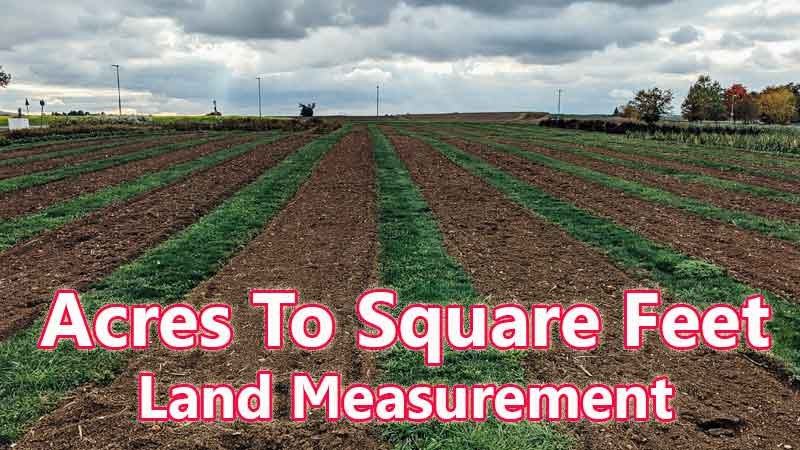# Acres To Square Feet Converter

Acres is a traditional land unit used to measure the land Area standardly. Here we are using the acres to square feet unit value conversion. And acres also comes under these land area units like Gonda, Kani, and square feet. In West Bengal, One acres is directly equivalent to 43560 square feet.

Input Here

acres

Output

square feet

1 Acres = 43560 Square Feet

## Measurement

Acres means - a unit of land measurement. This tool provides great calculation over acres to square feet, 1 acres to square feet, 1 acres in square feet, 1 acres equal to and etc. To convert acres to square feet, then multiply the unit value by 43560. Then, 1 acres * 43560 = 43560 square feet. Hence, 1 acres is equal to 43560 square feet.

square feet also comes under this land measurement unit and we are comparing values between acres traditional unit with other side land measurement unit.

## Formula For acres to square feet Conversion(acres to square feet formula)

The base formula for this acres to square feet converter is

square feet = acres * 43560

## How many square feet in a acres?

Step 1: To Convert 1 acres to square feet

Step 2: Applying formula square feet = acres * 43560, (i.e) multiply the unit value by 43560.

Step 3: Then, square feet = 1 * 43560 = 43560.

Step 4: Hence, 1 acres is equal to 43560 square feet.Some acres to square feet Conversion Chart for your reference:

 1 acres = 43560 square feet 2 acres = 87120 square feet 3 acres = 130680 square feet 4 acres = 174240 square feet 5 acres = 217800 square feet 6 acres = 261360 square feet 7 acres = 304920 square feet 8 acres = 348480 square feet 9 acres = 392040 square feet 10 acres = 435600 square feet

The acres to square feet conversion chart is above listed for your reference. This chart, however, represents the simple math calculation involved in the acres to square feet convertion online.

For Example: How much is 1 acres to square feet

Solution:

= (acres * 43560)

= (1 x 43560)

= 43560 square feet

For Example: How much is 120 acres in square feet

Solution:

= (acres * 43560)

= (120 x 43560)

= 5227200 square feet

This formula provides an instant answer for all your questions / People also search:

• 1 acres to square feet
• 1 acres is equal to how many square feet
• Formula to convert acres to square feet online
• How to convert acres to square feet?
• How much square feet is equal to a one acres?
• How many square feet in acres?
• 1 acres equal to square feet